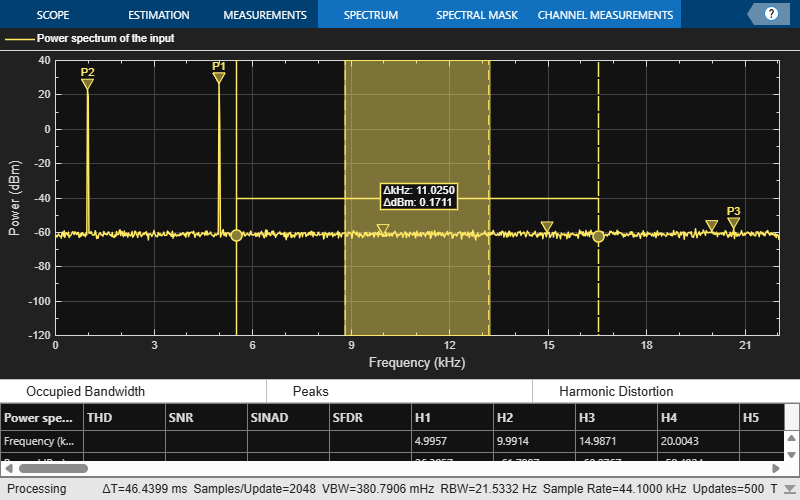# Obtain Measurements Data Programmatically for `spectrumAnalyzer` object

Compute and display the power spectrum of a noisy sinusoidal input signal using the `spectrumAnalyzer` MATLAB® object. Measure the peaks, cursor placements, adjacent channel power ratio, and distortion values in the spectrum by enabling these properties:

• `PeakFinder`

• `CursorMeasurements`

• `ChannelMeasurements`

• `DistortionMeasurements`

### Initialization

The input sine wave has two frequencies: 1000 Hz and 5000 Hz. Create two `dsp.SineWave` System objects to generate these two frequencies. Create a `spectrumAnalyzer` object to compute and display the power spectrum.

```Fs = 44100; Sineobject1 = dsp.SineWave(SamplesPerFrame=1024,PhaseOffset=10,... SampleRate=Fs,Frequency=1000); Sineobject2 = dsp.SineWave(SamplesPerFrame=1024,... SampleRate=Fs,Frequency=5000); SA = spectrumAnalyzer(SampleRate=Fs,SpectrumType="power",... PlotAsTwoSidedSpectrum=false,ChannelNames={'Power spectrum of the input'},... YLimits=[-120 40],ShowLegend=true); ```

### Enable Measurements Data

To obtain the measurements, set the `Enabled` property to `true`.

```SA.CursorMeasurements.Enabled = true; SA.ChannelMeasurements.Enabled = true; SA.PeakFinder.Enabled = true; SA.DistortionMeasurements.Enabled = true;```

### Use `getMeasurementsData`

Stream in the noisy sine wave input signal and estimate the power spectrum of the signal using the spectrum analyzer. Measure the characteristics of the spectrum. Use the `getMeasurementsData` function to obtain these measurements programmatically. The `isNewDataReady` function returns `true` when there is new spectrum data. Store the measured data in the variable `data`.

```data = []; for Iter = 1:1000 Sinewave1 = Sineobject1(); Sinewave2 = Sineobject2(); Input = Sinewave1 + Sinewave2; NoisyInput = Input + 0.001*randn(1024,1); SA(NoisyInput); if SA.isNewDataReady data = [data;getMeasurementsData(SA)]; end end```The right side of the spectrum analyzer shows the measurement panes you enabled. The values in these panes match the values in the last time step of the `data` variable. You can access the individual fields of `data` to obtain the various measurements programmatically.

### Compare Peak Values

Use the `PeakFinder` property to obtain peak values. Verify that the peak values in the last time step of `data` match the values shown on the spectrum analyzer plot.

`peakvalues = data.PeakFinder(end).Value `
```peakvalues = 3×1 26.9261 24.1149 -46.3163 ```
`frequencieskHz = data.PeakFinder(end).Frequency/1000`
```frequencieskHz = 3×1 4.9957 0.9905 0.0646 ```